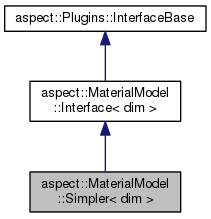ASPECT
aspect::MaterialModel::Simpler< dim > Class Template Reference
Inheritance diagram for aspect::MaterialModel::Simpler< dim >:[legend]

## Public Member Functions

bool is_compressible () const override

void evaluate (const MaterialModel::MaterialModelInputs< dim > &in, MaterialModel::MaterialModelOutputs< dim > &out) const overridePublic Member Functions inherited from aspect::MaterialModel::Interface< dim >
virtual ~Interface ()=default

virtual void initialize ()

virtual void update ()

virtual void create_additional_named_outputs (MaterialModelOutputs &outputs) const

virtual void fill_additional_material_model_inputs (MaterialModel::MaterialModelInputs< dim > &input, const LinearAlgebra::BlockVector &solution, const FEValuesBase< dim > &fe_values, const Introspection< dim > &introspection) const

const NonlinearDependence::ModelDependenceget_model_dependence () const

## Private Attributes

double k_value

Rheology::ConstantViscosity constant_rheology

EquationOfState::LinearizedIncompressible< dim > equation_of_state

## Functions used in dealing with run-time parameters

void parse_parameters (ParameterHandler &prm) override

static void declare_parameters (ParameterHandler &prm)Public Types inherited from aspect::MaterialModel::Interface< dim >
using MaterialModelInputs = MaterialModel::MaterialModelInputs< dim >

using MaterialModelOutputs = MaterialModel::MaterialModelOutputs< dim >Static Public Member Functions inherited from aspect::MaterialModel::Interface< dim >
static void declare_parameters (ParameterHandler &prm)Protected Attributes inherited from aspect::MaterialModel::Interface< dim >
NonlinearDependence::ModelDependence model_dependence

## Detailed Description

### template<int dim> class aspect::MaterialModel::Simpler< dim >

A material model that consists of globally constant values for all material parameters except the density, which depends linearly on the temperature. The model is considered incompressible.

This material model implements what the "Simple" model was originally intended to do, before it got too complicated.

Definition at line 45 of file simpler.h.

## § is_compressible()

template<int dim>
 bool aspect::MaterialModel::Simpler< dim >::is_compressible ( ) const
overridevirtual

Return whether the model is compressible or not. Incompressibility does not necessarily imply that the density is constant; rather, it may still depend on temperature or pressure. In the current context, compressibility means whether we should solve the continuity equation as $$\nabla \cdot (\rho \mathbf u)=0$$ (compressible Stokes) or as $$\nabla \cdot \mathbf{u}=0$$ (incompressible Stokes).

Implements aspect::MaterialModel::Interface< dim >.

## § evaluate()

template<int dim>
 void aspect::MaterialModel::Simpler< dim >::evaluate ( const MaterialModel::MaterialModelInputs< dim > & in, MaterialModel::MaterialModelOutputs< dim > & out ) const
overridevirtual

Function to compute the material properties in out given the inputs in in.

Implements aspect::MaterialModel::Interface< dim >.

## § declare_parameters()

template<int dim>
 static void aspect::MaterialModel::Simpler< dim >::declare_parameters ( ParameterHandler & prm )
static

Declare the parameters this class takes through input files.

## § parse_parameters()

template<int dim>
 void aspect::MaterialModel::Simpler< dim >::parse_parameters ( ParameterHandler & prm )
overridevirtual

Read the parameters this class declares from the parameter file.

Reimplemented from aspect::MaterialModel::Interface< dim >.

## § k_value

template<int dim>
 double aspect::MaterialModel::Simpler< dim >::k_value
private

Definition at line 77 of file simpler.h.

## § constant_rheology

template<int dim>
 Rheology::ConstantViscosity aspect::MaterialModel::Simpler< dim >::constant_rheology
private

Definition at line 79 of file simpler.h.

## § equation_of_state

template<int dim>
 EquationOfState::LinearizedIncompressible aspect::MaterialModel::Simpler< dim >::equation_of_state
private

Definition at line 80 of file simpler.h.

The documentation for this class was generated from the following file:
• /home/bob/source/include/aspect/material_model/simpler.h